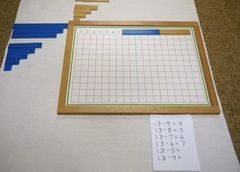# Difference between revisions of "Subtraction With Strip Board"

Subtraction With Strip Board
Math - Memory WorkLevel Primary
Age 5+

This activity gives the child practice figuring out and memorizing subtraction facts.

## Presentations

### Presentation One

1. Take the material to a mat or table. Lay out as shown.2. The first page should have just one problem: 18-9=
Take the 9 strip and cover up the numbers starting from the end. The last visible number is the answer.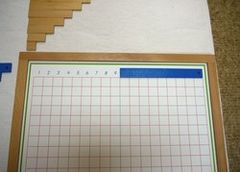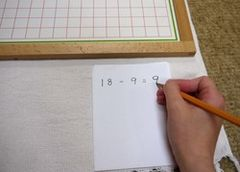3. The next page should have two problems: 17-9= and 17-8=
Lay the shortest of the plain wooden strips over the number 18 on the board.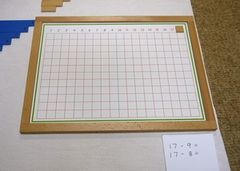4. Take the 9 strip and cover up the numbers starting from the end. The last visible number is the answer.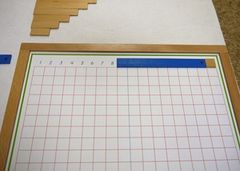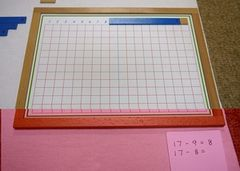5. Then take the 8 strip and cover up the numbers starting from the end. The last visible number is the answer.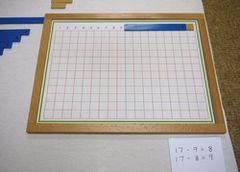6. next step